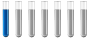## I don't understand this stoich problem ;-;

Chemistry and homework help forum.

Organic Chemistry, Analytical Chemistry, Biochemistry, Physical Chemistry, Computational Chemistry, Theoretical Chemistry, High School Chemistry, Colledge Chemistry and University Chemistry Forum.

Share your chemistry ideas, discuss chemical problems, ask for help with scientific chemistry questions, inspire others by your chemistry vision!

Please feel free to start a scientific chemistry discussion here!

Discuss chemistry homework problems with experts!

Ask for help with chemical questions and help others with your chemistry knowledge!

Moderators: Xen, expert, ChenBeier

MathematicalMistake
NewbiePosts: 4
Joined: Sun Oct 08, 2023 6:38 pm

### I don't understand this stoich problem ;-;

A 26.6 g sample of impure potassium nitrate (KNO3) was heated to complete decomposition according to the equation
2 KNO3(s) → 2 KNO2(s) + O2(g) .
After the reaction was complete, the solid residue (consisting of KNO2 and the original impurities) had a mass of 23.1 g. Assuming that only the potassium nitrate had decomposed, what was the percent KNO3 in the original sample?

I did this: (2 *(26.6 - 23.1)*101.103*100% ) / (32.00)(26.6)
ChenBeier
Distinguished MemberPosts: 1425
Joined: Wed Sep 27, 2017 7:25 am
Location: Berlin, Germany

### Re: I don't understand this stoich problem ;-;

I got the same amount.
3,5 g O2/ 32 g/ mol is 0,11 mol
This correspond to the double of KNO3 means 0,22 mol
Multiplied with molar mass 101 g/mol = 22,1 g
Percentage is 22,1/ 26,5 = 0,83 => 83%
MathematicalMistake
NewbiePosts: 4
Joined: Sun Oct 08, 2023 6:38 pm

### Re: I don't understand this stoich problem ;-;

Ok, thank you. I actually found a slight mistake in my method!